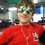# Sum of two algebraic integers is also an algebraic integer

How do you show that the sum of two algebraic integers is also an algebraic integer? Also, how do we find the minimal polynomial of the sum of the two algebraic integers given the minimal polynomials of both algebraic integers? For example, let $a=2+\sqrt{3}$ and $b=\sqrt{3}$ The minimal polynomial of $a$ is $x^2-4x+1$ and for $b$ is $x^3-3$, now how would we find the minimal polynomial of $a+b$ ? Furthermore, how would we prove that $a+b$ is also an algebraic integer?Note by Justin Stevens
6 years, 9 months ago

This discussion board is a place to discuss our Daily Challenges and the math and science related to those challenges. Explanations are more than just a solution — they should explain the steps and thinking strategies that you used to obtain the solution. Comments should further the discussion of math and science.

When posting on Brilliant:

• Use the emojis to react to an explanation, whether you're congratulating a job well done , or just really confused .
• Ask specific questions about the challenge or the steps in somebody's explanation. Well-posed questions can add a lot to the discussion, but posting "I don't understand!" doesn't help anyone.
• Try to contribute something new to the discussion, whether it is an extension, generalization or other idea related to the challenge.

MarkdownAppears as
*italics* or _italics_ italics
**bold** or __bold__ bold
- bulleted- list
• bulleted
• list
1. numbered2. list
1. numbered
2. list
Note: you must add a full line of space before and after lists for them to show up correctly
paragraph 1paragraph 2

paragraph 1

paragraph 2

[example link](https://brilliant.org)example link
> This is a quote
This is a quote
    # I indented these lines
# 4 spaces, and now they show
# up as a code block.

print "hello world"
# I indented these lines
# 4 spaces, and now they show
# up as a code block.

print "hello world"
MathAppears as
Remember to wrap math in $$ ... $$ or $ ... $ to ensure proper formatting.
2 \times 3 $2 \times 3$
2^{34} $2^{34}$
a_{i-1} $a_{i-1}$
\frac{2}{3} $\frac{2}{3}$
\sqrt{2} $\sqrt{2}$
\sum_{i=1}^3 $\sum_{i=1}^3$
\sin \theta $\sin \theta$
\boxed{123} $\boxed{123}$

Sort by:

It is easier to prove that $a+b$ is an algebraic integer. Let $f(x)$ be a (not necessarily minimal) integer polynomial such that $f(a) = 0$, with roots $a_i$. Let $g(x)$ be a (not necessarily minimal) integer polynomial such that $g(b) = 0$, with roots $b_j$. Then, consider the polynomial

$h(x) = \prod ( x - a_i - b_j)$

It remains to show that the coefficients are integers. If you expand it, you can use Veita's formulas (though tediously). This is motivated from Galois theory which tells us that the algebraic integers form a ring.

The reason why $h(x)$ need not be the minimal polynomial, is because things might cancel out. For example, if $a = \sqrt{2}$ with minimal polynomial $x^2 - 2$ and $b = \sqrt{2}$ with minimal polynomial $x^2 -2$, then the above construction gives us $h(x) = x^4 - 4 x^2$, while $a+b= 0$ which has minimal polynomial of $x$. I don't think a general solution exists, but I might be wrong.

- 6 years, 9 months ago

This keeps only proves that $a + b$ is an algebraic number because $h$ is not necessarily an integer polynomial. This is because the symmetric sums of $a_i, b_i$ are rational from Vieta, not integral. If $f, g$ were monic, then this would be entirely correct.

- 6 years, 9 months ago

The definition of an algebraic integer, is that it is a root of a monic polynomial. I should have mentioned that, as it wasn't stated in the post.

Of course, there are many other ways of understanding algebraic integers, especially if you have a knowledge of field theory / abstract algebra. I wasn't certain how much he knew, and I opted to go for the most basic (and crude / brute force) approach. For example, if you used the definition that $\alpha$ is an algebraic integer if $\mathbb{Z} [\alpha]$ is a finitely generated $\mathbb{Z} -$module, the result is immediate. However, that wouldn't have been helpful to many people.

- 6 years, 9 months ago

Thanks for the solution! Can you elaborate on the use of Vieta's formulas to prove that all the coefficients of $h(x)$ are integers?

- 6 years, 9 months ago

http://www.incertia.net/blog/some-proofs-regarding-symmetric-polynomials-and-algebraic-numbers/

- 6 years, 9 months ago

We can use the fundamental theorem of symmetric polynomial with the same polynomial defined by chung repeatedly to prove in general.

- 3 years, 6 months ago